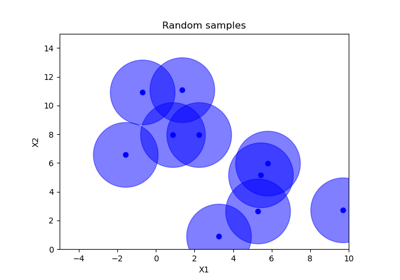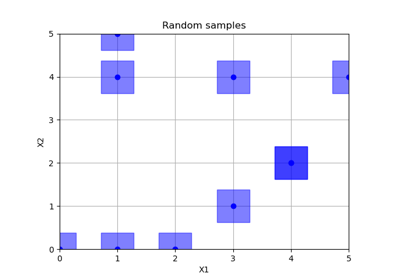# skopt.sampler.Sobol¶

class skopt.sampler.Sobol(min_skip=0, max_skip=1000, randomize=False)[source][source]

Generates a new quasirandom Sobol vector with each call.

The routine adapts the ideas of Antonov and Saleev.

Parameters
min_skipint

minimum skipped seed number. When min_skip != max_skip a random number is picked.

max_skipint

maximum skipped seed number. When min_skip != max_skip a random number is picked.

randomizebool, default=False

When set to True, random shift is applied

References

Antonov, Saleev, USSR Computational Mathematics and Mathematical Physics, Volume 19, 1980, pages 252 - 256.

Paul Bratley, Bennett Fox, Algorithm 659: Implementing Sobol’s Quasirandom Sequence Generator, ACM Transactions on Mathematical Software, Volume 14, Number 1, pages 88-100, 1988.

Bennett Fox, Algorithm 647: Implementation and Relative Efficiency of Quasirandom Sequence Generators, ACM Transactions on Mathematical Software, Volume 12, Number 4, pages 362-376, 1986.

Ilya Sobol, USSR Computational Mathematics and Mathematical Physics, Volume 16, pages 236-242, 1977.

Ilya Sobol, Levitan, The Production of Points Uniformly Distributed in a Multidimensional Cube (in Russian), Preprint IPM Akad. Nauk SSSR, Number 40, Moscow 1976.

Methods

 generate(dimensions, n_samples[, random_state]) Creates samples from Sobol set. set_params(**params) Set the parameters of this initial point generator.
 init
__init__(min_skip=0, max_skip=1000, randomize=False)[source][source]

Initialize self. See help(type(self)) for accurate signature.

generate(dimensions, n_samples, random_state=None)[source][source]

Creates samples from Sobol set.

Parameters
dimensionslist, shape (n_dims,)

List of search space dimensions. Each search dimension can be defined either as

• a (lower_bound, upper_bound) tuple (for Real or Integer dimensions),

• a (lower_bound, upper_bound, "prior") tuple (for Real dimensions),

• as a list of categories (for Categorical dimensions), or

• an instance of a Dimension object (Real, Integer or Categorical).

n_samplesint

The order of the Sobol sequence. Defines the number of samples.

random_stateint, RandomState instance, or None (default)

Set random state to something other than None for reproducible results.

Returns
np.array, shape=(n_dim, n_samples)

Sobol set

set_params(**params)[source]

Set the parameters of this initial point generator.

Parameters
**paramsdict

Generator parameters.

Returns
——-
selfobject

Generator instance.

## Examples using skopt.sampler.Sobol¶